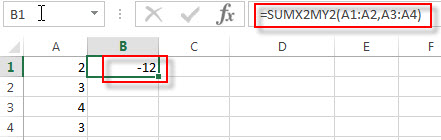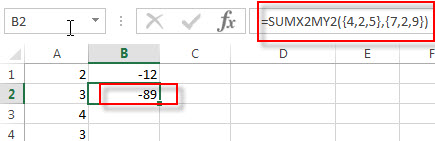# Excel SUMX2MY2 Function

This post will guide you how to use Excel SUMX2MY2 function with syntax and examples in Microsoft excel.

### Description

The Excel SUMX2MY2 function returns the sum of the difference of squares between two supplied arrays.

The SUMX2MY2 function is a build-in function in Microsoft Excel and it is categorized as a Math and Trigonometry Function.

The SUMX2MY2 function is available in Excel 2016, Excel 2013, Excel 2010, Excel 2007, Excel 2011 for Mac.

### Syntax

The syntax of the SUMX2MY2 function is as below:

`=SUMX2MY2 (array_x, array_y)`

Where the SUMX2MY2 function arguments are:

• array_x -This is a required argument. The first array or range of values.
• array_y -This is a required argument. The second array or range of values

Note:

• The arguments can be numbers or names, arrays or references that contain numbers.
• Empty cells, logical values, text or error values in the array or reference will be ignored.
• If array_x and array_y arguments have different lengths, the Function will return #N/A Error.
• The equation for the sum of the difference of squares is:

### Excel SUMX2MY2 Function Examples

The below examples will show you how to use Excel SUMX2MY2 Function to sum the differentce of squares of two given array values in Excel.

1# to sum of the difference of squares of the two arrays A1:A2 and A3:A4 , enter the following formula in Cell B1.

`=SUMX2MY2(A1:A2,A3:A4)`2# tosum of the difference of squares of the two arrays constants {4,2,5} and {7,2,9}, enter the following formula in Cell B2.

`=SUMX2MY2({4,2,5},{7,2,9})`### Related Functions

• Excel SUM Function
The Excel SUM function will adds all numbers in a range of cells and returns the sum of these values. You can add individual values, cell references or ranges in excel.The syntax of the SUM function is as below:= SUM(number1,[number2],…)…
• Excel SUMIF Function
The Excel SUMIF function sum the numbers in the range of cells that meet a single criteria that you specify. The syntax of the SUMIF function is as below:=SUMIF (range, criteria, [sum_range])…
• Excel SUMIFS Function
The Excel SUMIFS function sum the numbers in the range of cells that meet a single or multiple criteria that you specify. The syntax of the SUMIFS function is as below:=SUMIFS (sum_range, criteria_range1, criteria1, [criteria_range2, criteria2], …)…
• Excel SUMSQ Function
The Excel SUMSQ function returns the sum of squares of a given set of arguments.The syntax of the SUMSQ function is as below:=SUMSQ(number1, [number2], …)…